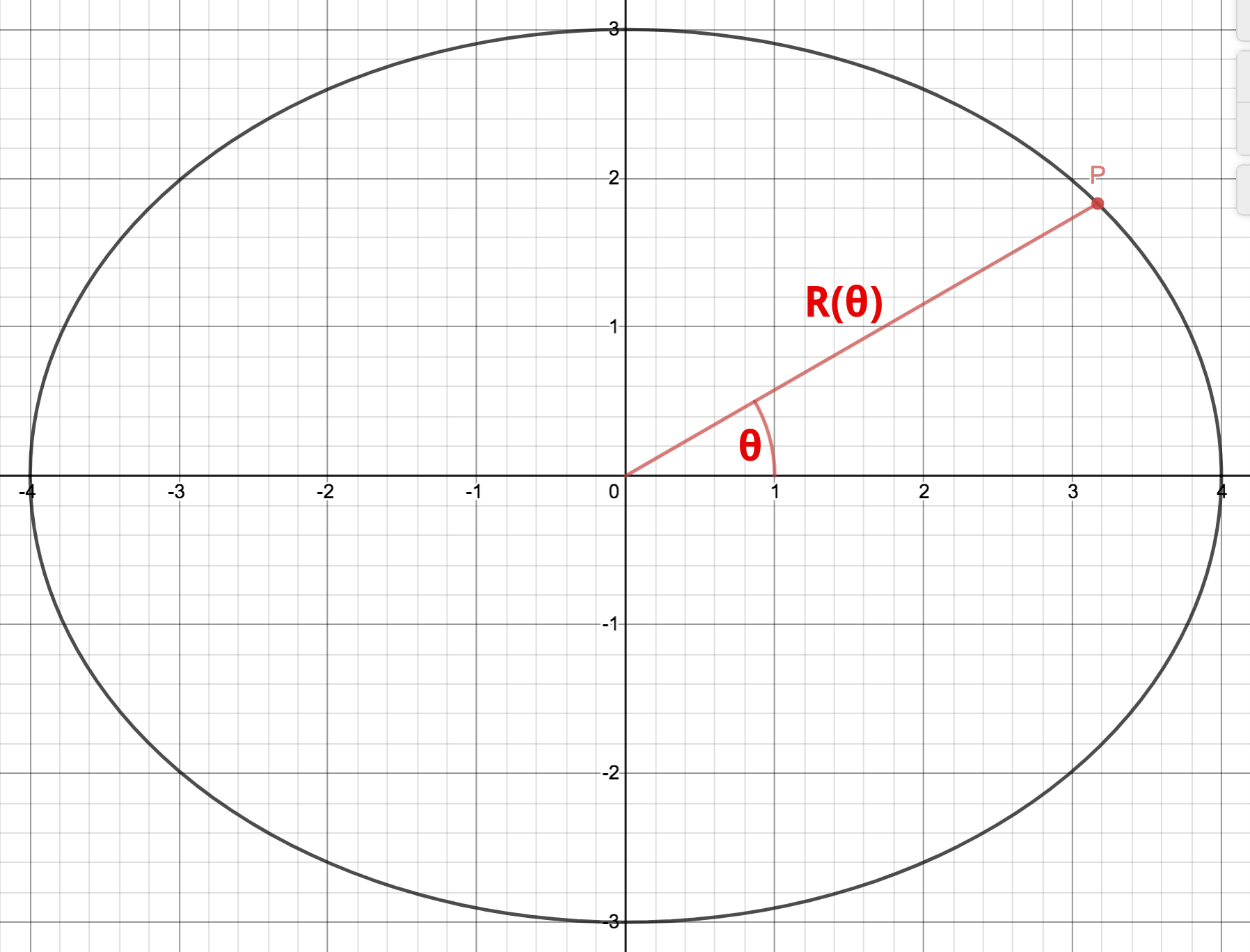TL;DR

Don’t even get me started on ellipses in SVG.

After tacking the bounding boxes for segments and Bézier curves, our last path section type is the ellipse (well, an arc of ellipse, actually). Before going to the code, we have to take a look at the math though, and we’ll start here.

Let’s start saying that it’s addressed in the proposed SVG2 specification, in particular Appendix B. So, you actually don’t need to look any further for the maths, unless you want to understand it.

There can be multiple ways to represent an ellipse, and it’s convenient to know them. It’s useful to remember that an ellipse is a sort of a circle, but instead of having one single radius, it has two: one in the $X$ direction, another one in the $Y$ direction. We will call them $r_x$ and $r_y$.

I now this is pretty vague at this point, but it will become clearer when we will look at the role that these two parameters play in the equations.

Note: we will only consider ellipses in the Real plane.

# Implicit representation

An ellipse centered in the origin can be described as the set of points $\mathbf{P} = (P_x, P_y) = (x, y)$ that satisfy the following equation:

$\left(\frac{x}{r_x}\right)^2 + \left(\frac{y}{r_y}\right)^2 = 1\\ x \in \mathbb{R}, y \in \mathbb{R}$

In a sense, this is the equation of a unitary circle in the plane obtained by properly stretching the two coordinates according to $r_x$ and $r_y$, which is why they are called radii.

This equation can be easily generalized to set its center on an arbitrary point $\mathbf{C} = (C_x, C_y)$ of the plane:

$\left(\frac{x - C_x}{r_x}\right)^2 + \left(\frac{y - C_y}{r_y}\right)^2 = 1\\$

We might also want to see how the equations change when rotating the ellipse, but we will not go down this path here.

# Explicit representation

The implicit equation in the previous section can be used to get an explicit form where we can express $y$ as a function of $x$. Well, actually as two functions of $x$, because the squaring is equally satisfied both by positive and negative values for $y$:

$y = C_y + \pm \frac{r_y}{r_x} \cdot \sqrt{r_x^2 - (x - C_x)^2} \\ x \in [-r_x, r_x]$

# Parametric representation

Both coordinates for a point on the ellipse (centered on the origin) can be expressed in terms of a third independent parameter $t$ as follows:

$P_x(t) = x(t) = r_x \cdot cos(t) \\ P_y(t) = y(t) = r_y \cdot sin(t) \\ t \in [0, 2\pi[$

While $t$ can be considered an angle, it is not the angle between the vector $\mathbf{v} = \mathbf{P} - \mathbf{O} = (x(t), y(t))$ (with $\mathbf{O}$ representing the origin of the coordinate axes) and the unitary vector of the $X$ axis (i.e. vector $(1, 0)$). To see this, let’s consider the following figure, that shows the polar representation for the point $\mathbf{P}$:The graphic was produced online at desmos.com, with some additional labeling (see original here).

We can easily see that:

$P_x(t) = x(t) = r_x \cdot cos(t) = R(\theta) \cdot cos(\theta) \\ P_y(t) = y(t) = r_y \cdot sin(t) = R(\theta) \cdot sin(\theta) \\ t \in [0, 2\pi[, \theta \in [0, 2\pi[$

so, dividing the two equations:

$\frac{r_y}{r_y} \cdot tan(t) = tan(\theta) \\$

i.e. $t$ is not the same as $\theta$ for a non-circular ellipse (that is, one where $r_x \ne r_y$).

As a matter of fact, remember that the ellipse is actually a unit circle that is enlarged/squashed differently in the $X$ and $Y$ directions thanks to $r_x$ and $r_y$? The parameter $t$ happens to be the angle of the image of point $\mathbf{P}$ on that unit circle (this is why parameters $\theta_1$ and $\theta_2$ in Appendix B are defined as […]angle of the elliptical arc prior to the stretch […]).

It’s easy to move the ellipse’s center to an arbitrary point $\mathbf{C} = (C_x, C_y)$ and rotate the ellipse counter-clockwise by an angle $\phi$:

$x(t) = C_x + cos(\phi) \cdot r_x \cdot cos(t) - sin(\phi) \cdot r_y \cdot sin(t) \\ y(t) = C_y + sin(\phi) \cdot r_x \cdot cos(t) + cos(\phi) \cdot r_y \cdot sin(t)$

# Enough for now…

We’ve seen three ways of representing an ellipse on the $XY$ plane… shall we call it a day?

Comments? Octodon, , GitHub, Reddit, or drop me a line!